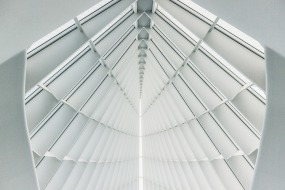## Unit 1: To Understand is to Perceive Patterns

### Overview

In this unit, you will be looking at patterns and relationships and the multiple ways of representing the relationships. You will focus on the characteristics of linear and non-linear relations including identifying the key characteristics and features of these relations and using these characteristics and features to connect different representations of the same relation.

You will also learn to identify, select and use the mathematical processes.## Unit 2: Distinguishing Patterns by Relationships

### Overview

In this unit, you will extend your understanding of linear and non-linear relations. You will focus on vocabulary, various representations for the relation and the connections between relations. You will:

• describe linear relations using graphs, equations and precise vocabulary and connect the slope to other topics;
• describe quadratic relations using graphs and new vocabulary;
• describe ratios of side lengths in triangles using new definitions;
• determine surface area of a pyramid You will continue to identify and use the mathematical processes.## Unit 3: Algebraic Equations Can Be Expressed in Equivalent Ways

### Overview

In this unit, you will learn different ways to write algebraic expressions, and how to change from one form of expression to another. You will be able to show relationships using graphs, algebra and words. You will learn to recognize which form of expression is best in different situations, and this will help you to solve problems. You will develop these skills working with different types of algebraic expressions, including those relating to linear relations, quadratic relations and relations with triangles.

## Unit 4: Using Our Skills to Solve Problems

### Overview

In this unit, you will have the opportunity to use linear relations, quadratic relations, trigonometric relations and the relations of 2D and 3D shapes and figures to solve a variety of problems. After reading the problem and determining the given information and the information required, you will create a plan that you will use to solve the problem. Once the problem is solved, you will reflect on your solution and consider other ways that you could solve similar problems in the future.## Unit 5: Culminating Activity

### Overview

This is the culminating activity for the course. You will solve problems that require knowledge of and skill with linear relations, quadratic relations, trigonometric relations and relations of 2D and 3D shapes and figures.

### Unit 5 Activities

Activity 1: Celebration